# 第3次作业

### 作业要求一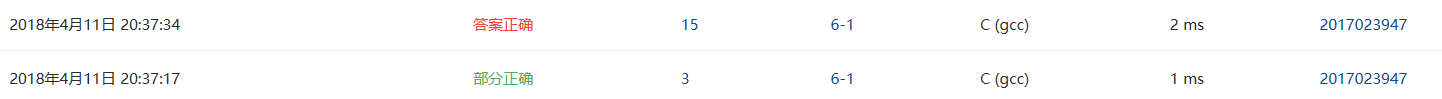1.设计思路

(1)主要描述题目算法。可以用文字描述（不允许使用伪代码），如：
第一步：定义月份对应的指针数组与整形变量i
第二步：用for循环遍历数组
第三步：if条件语句用来判断不同条件下的返回值并输出

（2）流程图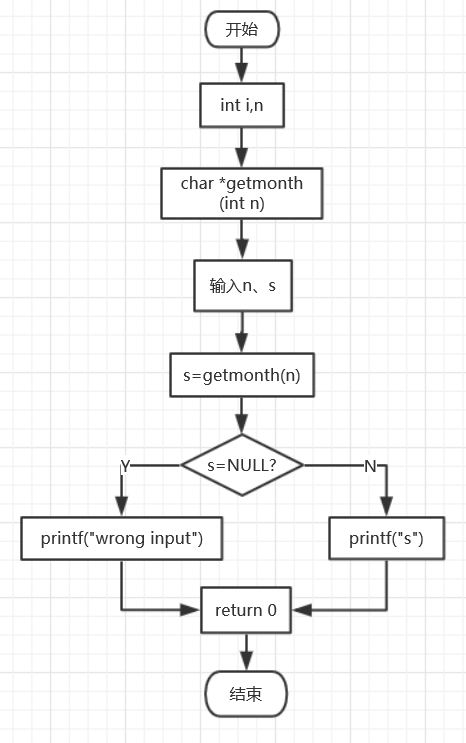2.实验代码

char *getmonth( int n )
{
char *month={"January","February","March","April","May","June","July","August","September","October","November","December"};
int i=0;
for(i=0;i<13;i++)
{
if(i==0)
{
continue;
}else if(n==i)
{
return *(month+i-1);
}
}
if(n>=13||n<=0)
{
return NULL;
}
} 

3.本题调试过程碰到问题及解决办法

错误信息：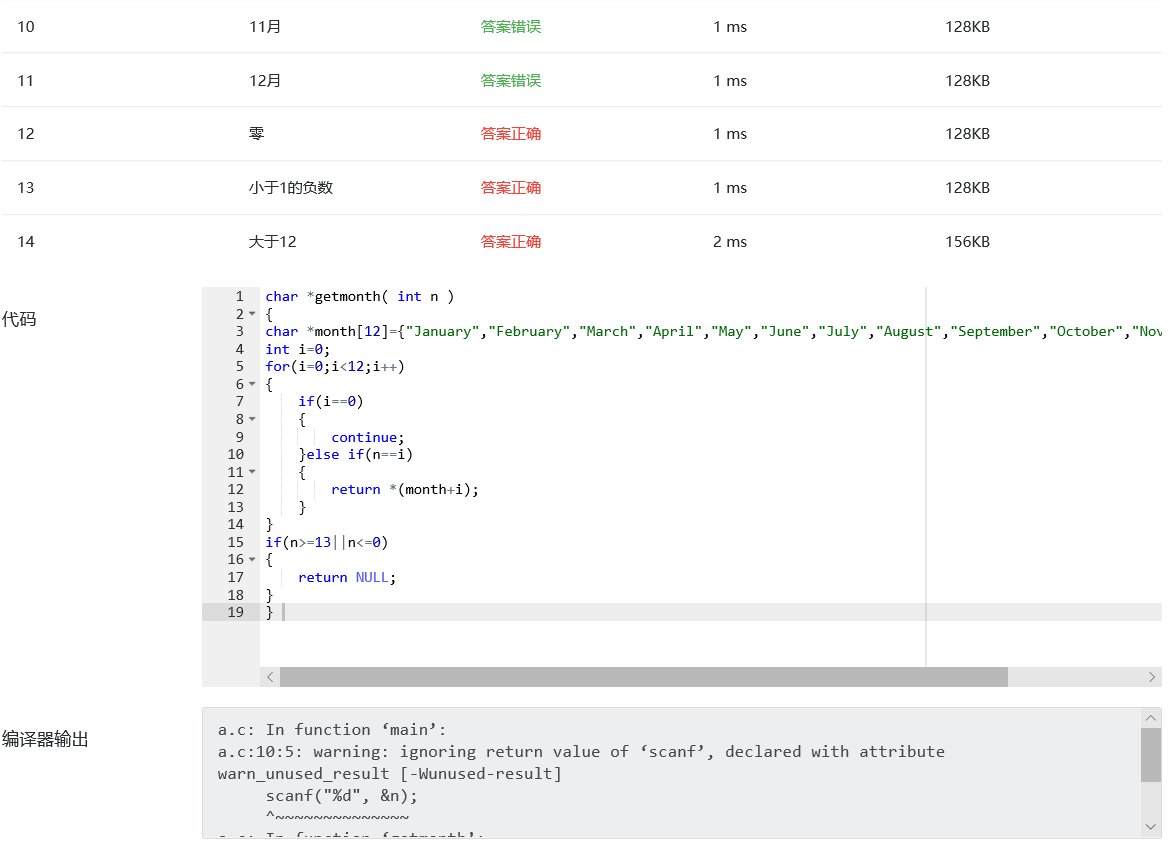错误原因：n=i时的返回值取地址错误
改正方法：改为*(month+i-1)即可，为第n-1行首元素的地址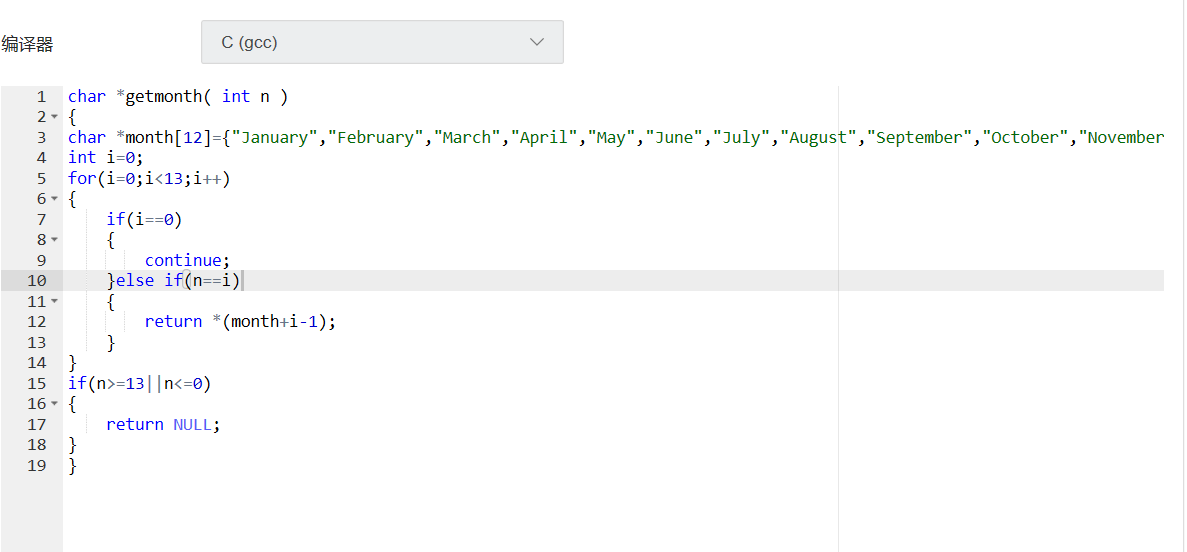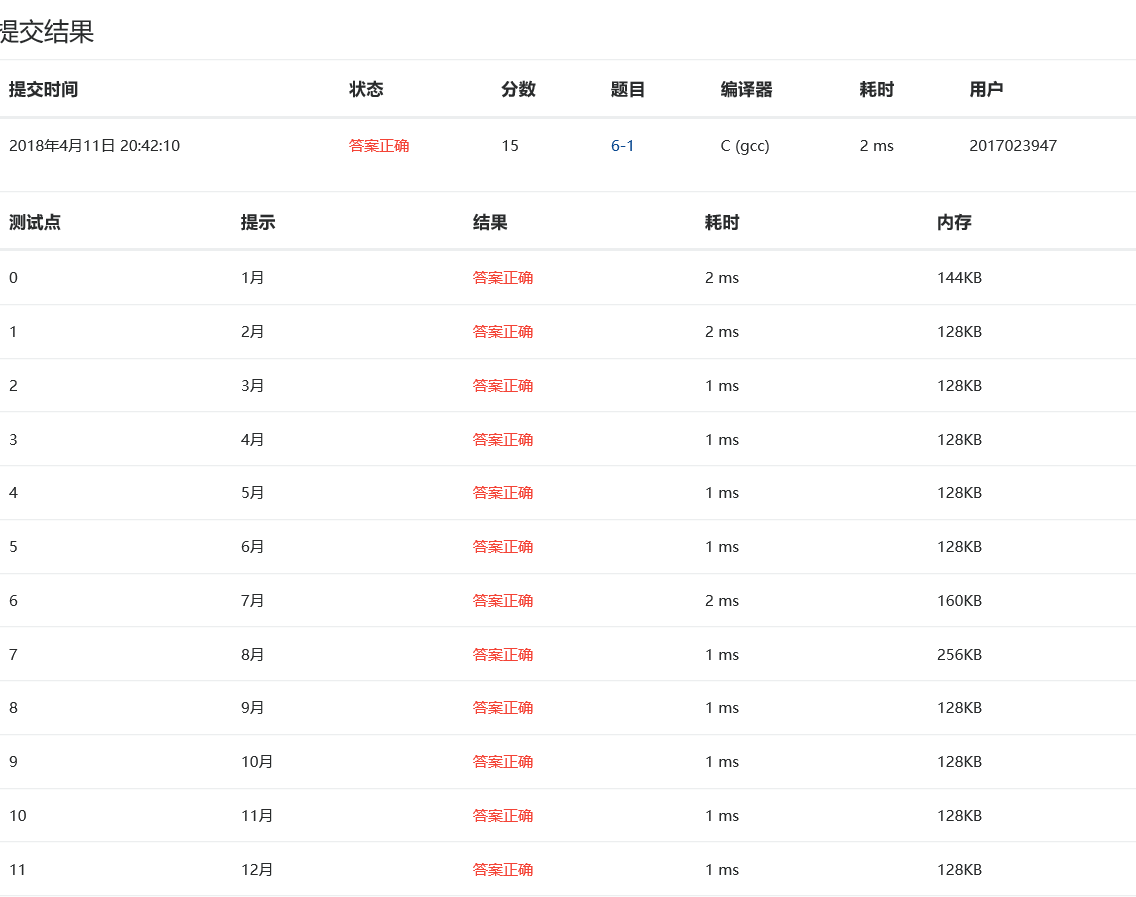6-2 查找星期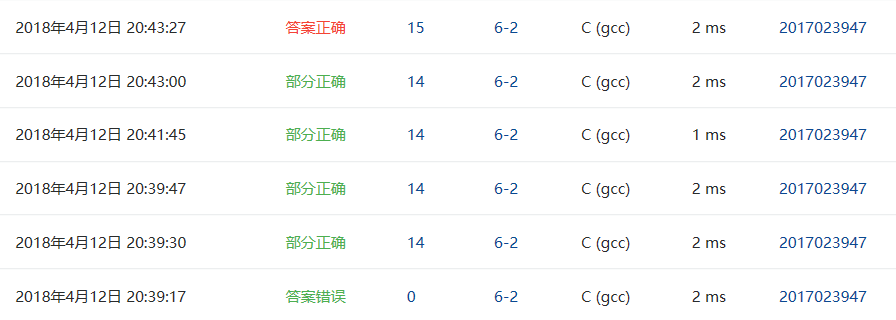1.设计思路
(1)主要描述题目算法。可以用文字描述（不允许使用伪代码），
第一步：定义整形变量 i 和星期对应的指针数组
第二步：利用for循环遍历数组
第三步：用if语句与strcmp函数进行分类讨论，当查找到相应元素时返回整形变量值

2.实验代码

int getindex( char *s )
{
int i;
char *week= { "Sunday","Monday","Tuesday","Wednesday","Thursday","Friday","Saturday" };
for (i = 0; i < 7; i++)
{
if (strcmp(s, week[i]) == 0)
{
break;
}
}
if (i==7)
{
i = -1;
}
return i;
}

3.本题调试过程碰到问题及解决办法

错误信息：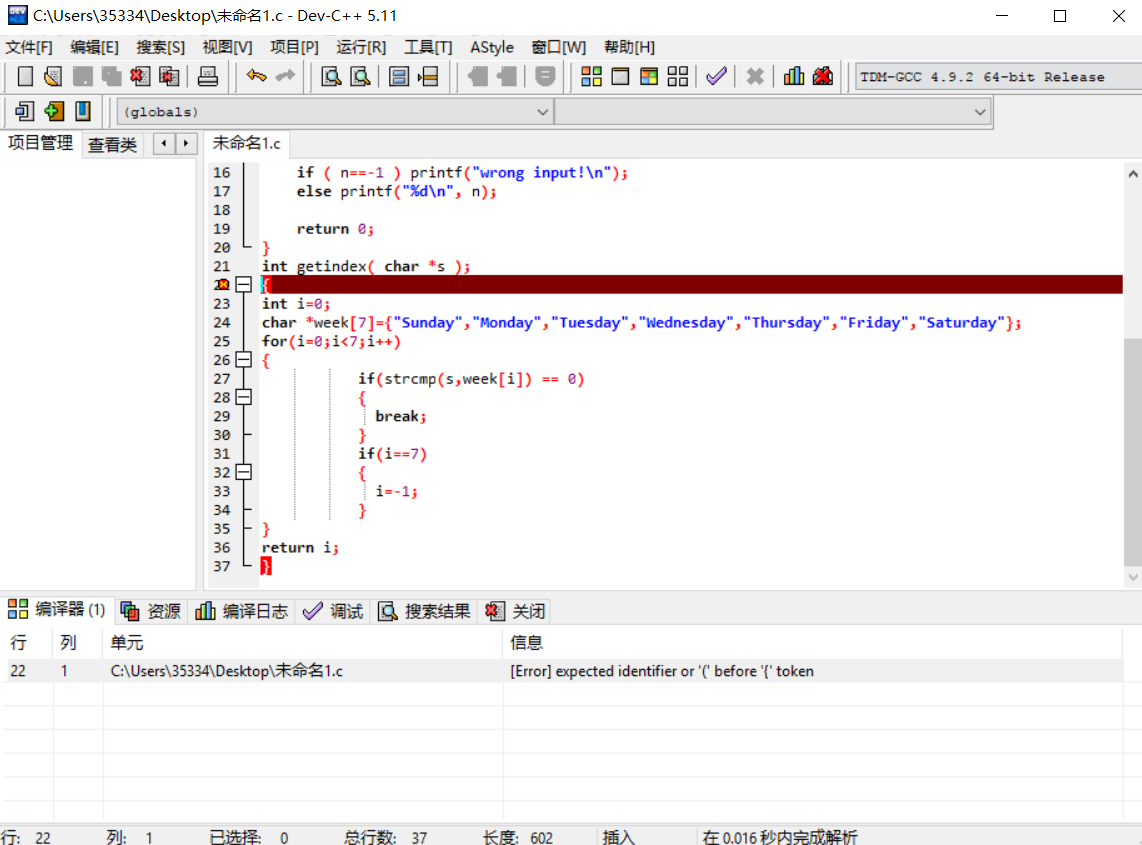错误原因：输入函数接口定义时在后方加了多余的分号" ; "
改正方法：通过dev-c++提醒找出，低级错误6-3 计算最长的字符串长度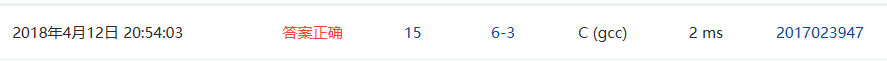1.设计思路
(1)主要描述题目算法。可以用文字描述（不允许使用伪代码），
第一步：定义两个整形变量 i，l
第二步：用for循环遍历数组
第三步：if语句中调用strlen函数进行计算并比较，最后返回 l 值

2.实验代码

int max_len( char *s[], int n )
{
int i,l=0;
for(i=0;i<n;i++)
{
if(l<strlen(*(s+i)))
{
l=strlen(*(s+i));
}
}
return l;
}

3.本题调试过程碰到问题及解决办法

错误信息：无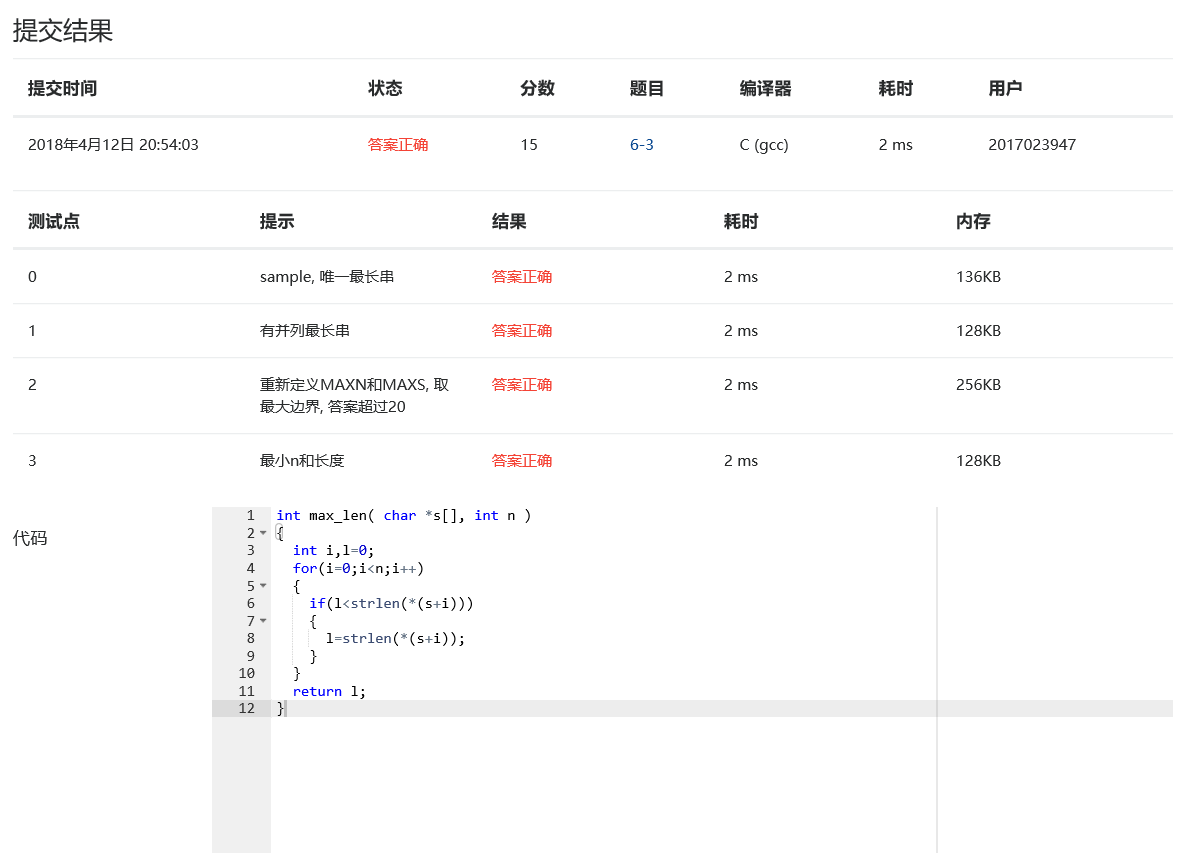6-4 指定位置输出字符串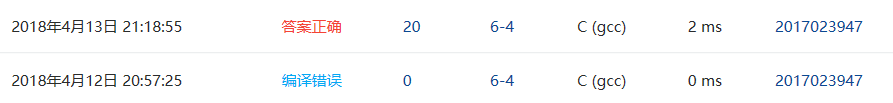1.设计思路
(1)主要描述题目算法。可以用文字描述（不允许使用伪代码），
第一步：定义整形变量i，j，通过一个for循环将ch1的值赋给*（s+i）
第二步：设计一个指针变量，判断*(s+j)!的值

第三步：整体遍历后，输出并返回（s+i）的值

2.实验代码

char *match( char *s, char ch1, char ch2 ){
int i,j;
for(i=0;*(s+i)!='\0';i++)
{
if(*(s+i)==ch1)
{
char *a=  &s[i];
for(j=i;(*(s+j)!=ch2)&&(*(s+j)!='\0');j++)
{
printf("%c",*(s+j));
}
if(*(s+j)!='\0')
printf("%c",*(s+j));
printf("\n");
return a;
}
}
printf("\n");
return s+i;
}

3.本题调试过程碰到问题及解决办法

错误信息：错误原因：函数接口定义编写错误，导致代码无法正常编译
改正方法：改变思路并改正定义，用一个for循环和if语句进行遍历和输出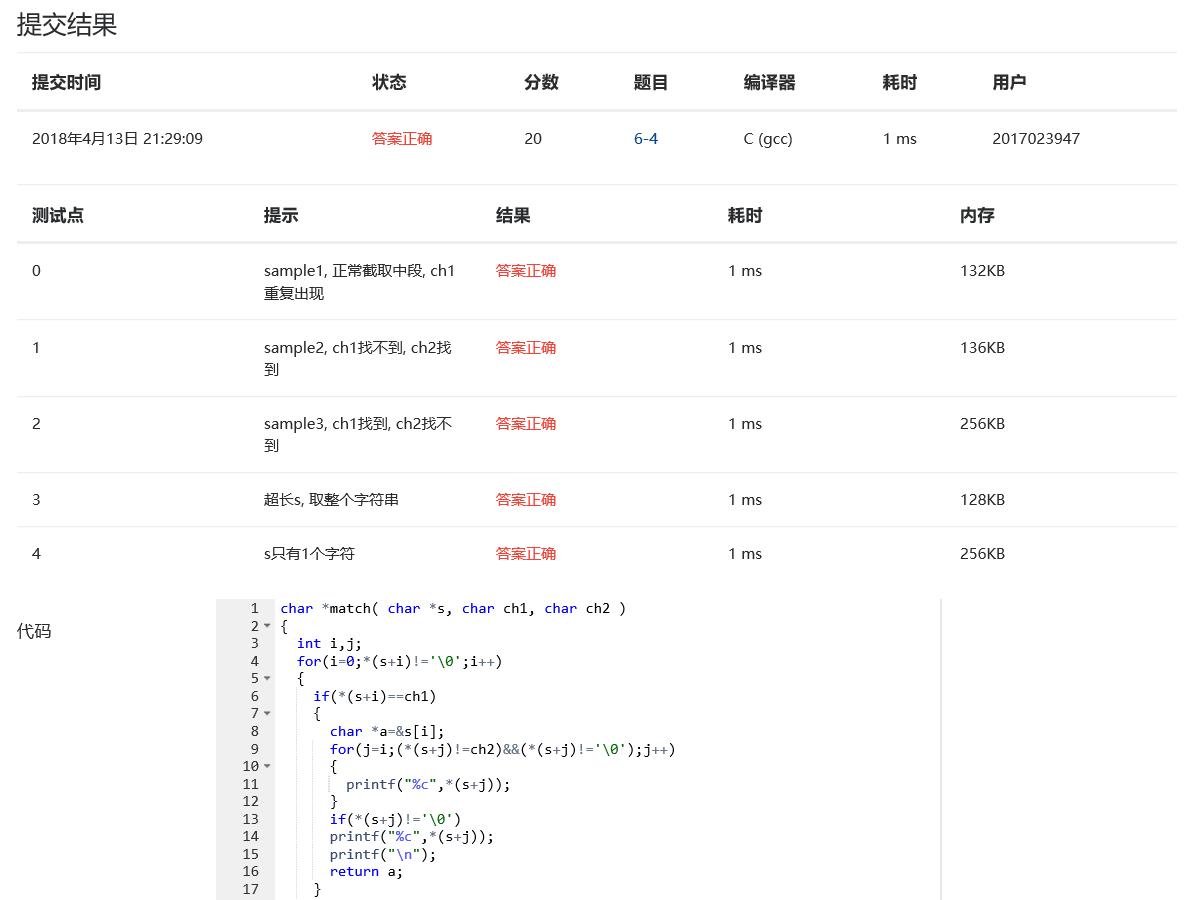2）一道编程题：

#include <stdio.h>
int main ()
{
int a=201,b=3947;
int *p = (int *)malloc((a*b) *sizeof(int));
int *q = (int *)malloc((a*b) *sizeof(int));
int i=0,j;
for(i=0;i<(a*b);i++)
{
p[i] = i+1;
}
for(i=0;i<(a*b);i++)
{
for(j = i+1;j<=(a*b);j++)
{
if((p[i] !=1) && ( p[j] != 1) ){
if(p[j]%p[i] ==0)
{
p[j] = 1;
}
}
}
}
j=0;
for(i=0;i<(a*b);i++)
{
if(p[i] != 1)
{
printf(" %d",p[i]);
j++;
}
if(j == 5)
{
printf("\n");
j=0;
}
}
}

6-1 奇数值结点链表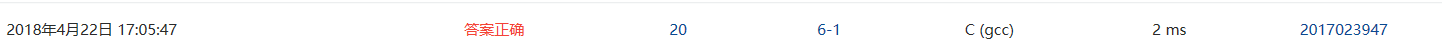1.设计思路
(1)主要描述题目算法。可以用文字描述（不允许使用伪代码），
第二步：在getodd函数中，根据题目要求的条件对链表结点中date的值进行分类判断。
第三步：需考虑若最后新建链表有可能为空的情况，最后按照要求返回要求链表的头结点。

（2）流程图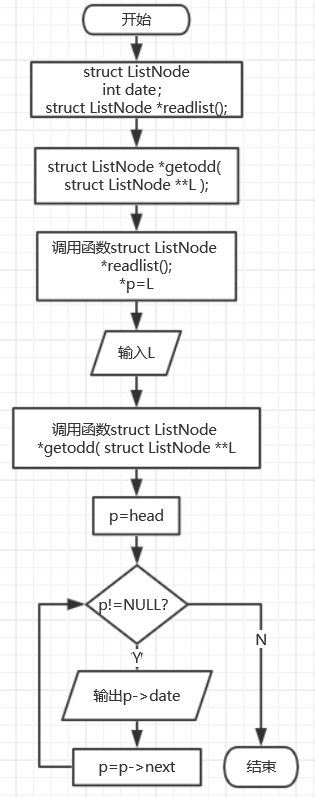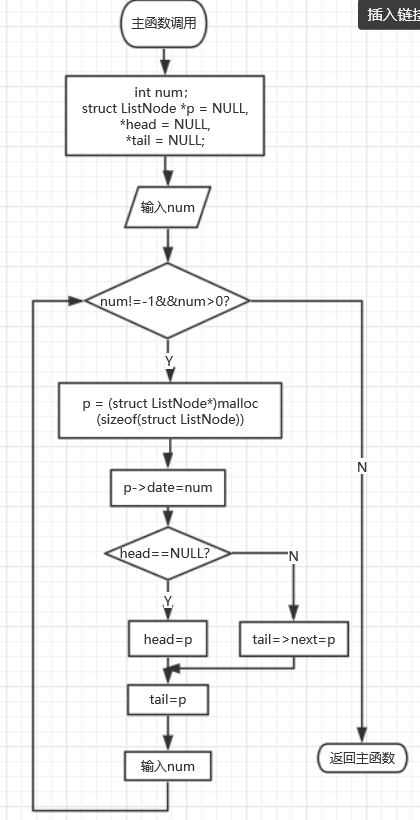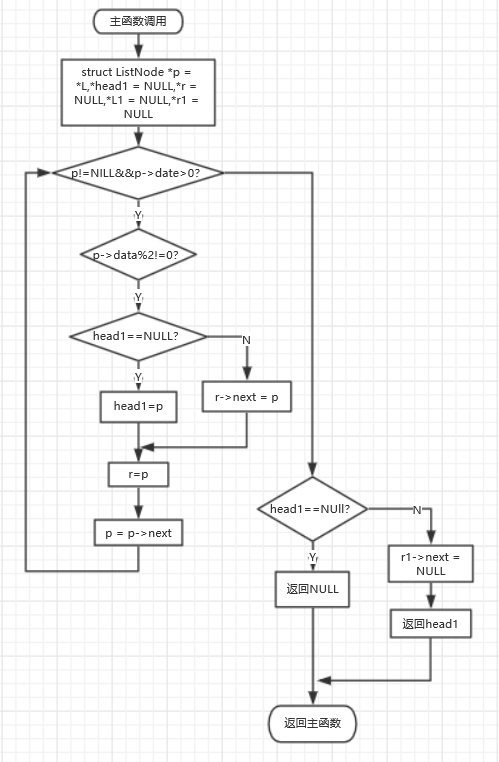2.实验代码

struct ListNode *readlist() {
int n;
struct ListNode *p = NULL,*head = NULL,*tail = NULL;
scanf("%d",&n);
while(n!=-1 && n>0 )
{
p = (struct ListNode*)malloc(sizeof(struct ListNode));
p->data = n;
{
} else
{
tail->next = p;
}
tail = p;
scanf("%d",&n);
}
{
return NULL;
}
tail->next = NULL;
}
struct ListNode *getodd( struct ListNode **L )
{
struct ListNode *p = *L,*head1 = NULL,*r = NULL,*L1 = NULL,*r1 = NULL;
while(p!=NULL&&p->data>0)
{
if(p->data%2!=0)
{
{
} else
{
r->next = p;
}
r = p;
} else
{
if(L1 ==NULL)
{
L1 = p;
} else
{
r1->next = p;
}
r1 = p;
}
p = p->next;
}
{
return NULL;
} else
{
r->next = NULL;
}
if(L1==NULL)
{
*L = NULL;
} else
{
r1->next = NULL;
*L = L1;
}
}

3.本题调试过程碰到问题及解决办法

无。6-2 学生成绩链表处理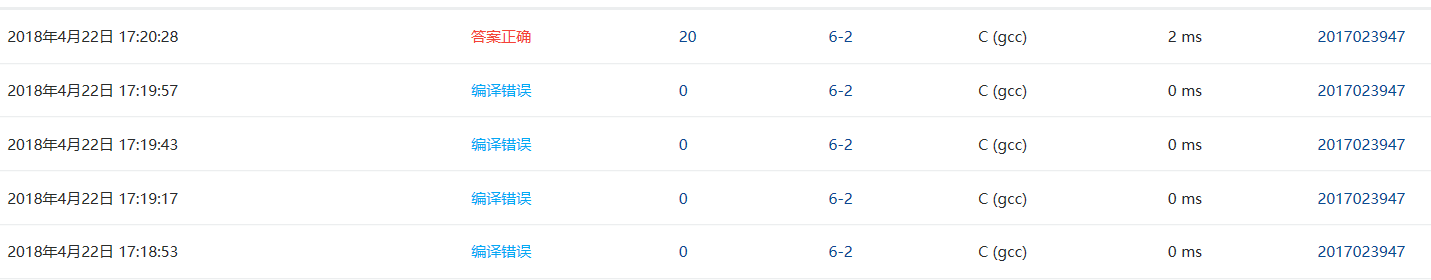1.设计思路
(1)主要描述题目算法。可以用文字描述（不允许使用伪代码），
第一步：在createlist函数中将输入的学号，姓名和分数存储到链表中
第二步：在deletelist函数中遍历链表的结点，若结点中的分数小于规定的值则释放此结点的内存，需注意若最终结点全被释放完内存，即返回的头结点为空的情况
第三步：将判断后链表的头结点返回主函数。

2.实验代码

struct stud_node *createlist() {
int num;
char name;
int score;

scanf("%d",&num);
while (num != 0)
{
p = (struct stud_node *)malloc (sizeof (struct stud_node));
scanf ("%s %d", p->name, &p->score);
p->num = num;

else
tail->next = p;
tail = p;
scanf ("%d", &num);
}
}
struct stud_node *deletelist( struct stud_node *head, int min_score ) {
while(L!=NULL) {
if(L->score>=min_score)
{
} else {
tail1->next = L;
}
tail1 = L;
}
L=L->next;
}
return NULL;
} else {
tail1->next =NULL;
}
} 

3.本题调试过程碰到问题及解决办法

错误信息：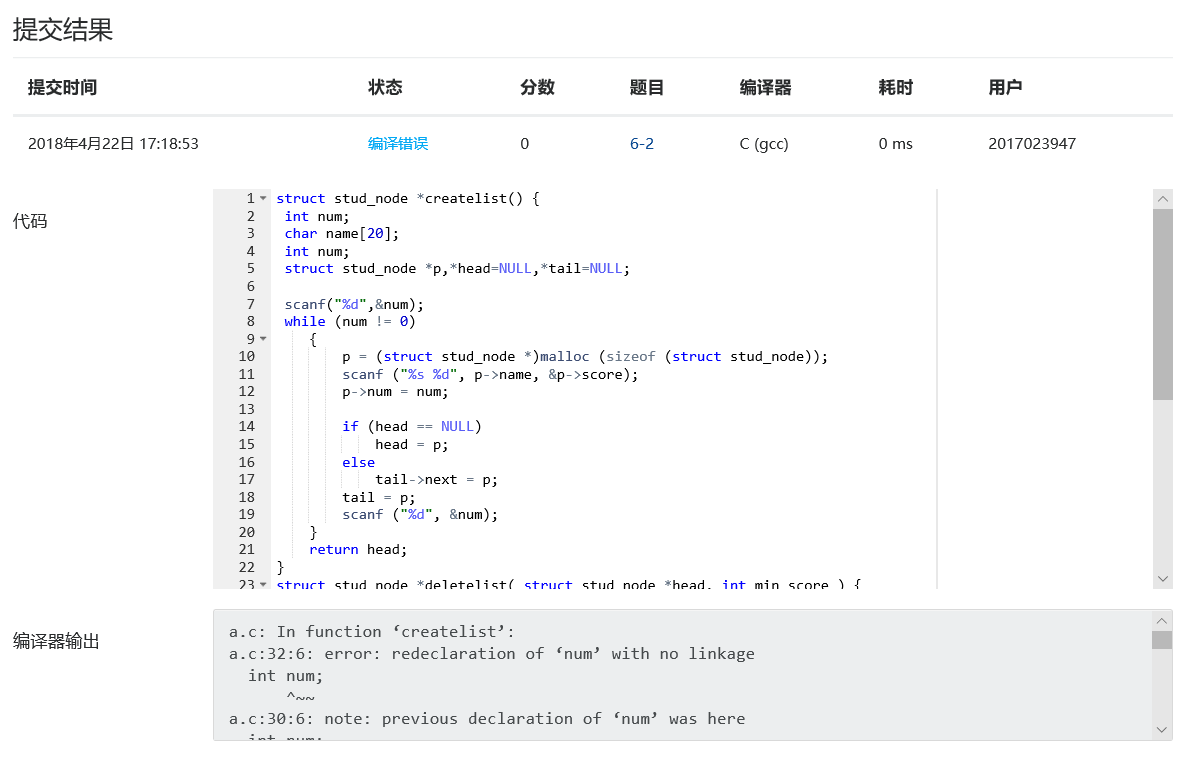错误原因：定义时未定义score而定义了两次num，低级错误
改正方法：重新定义整形变量score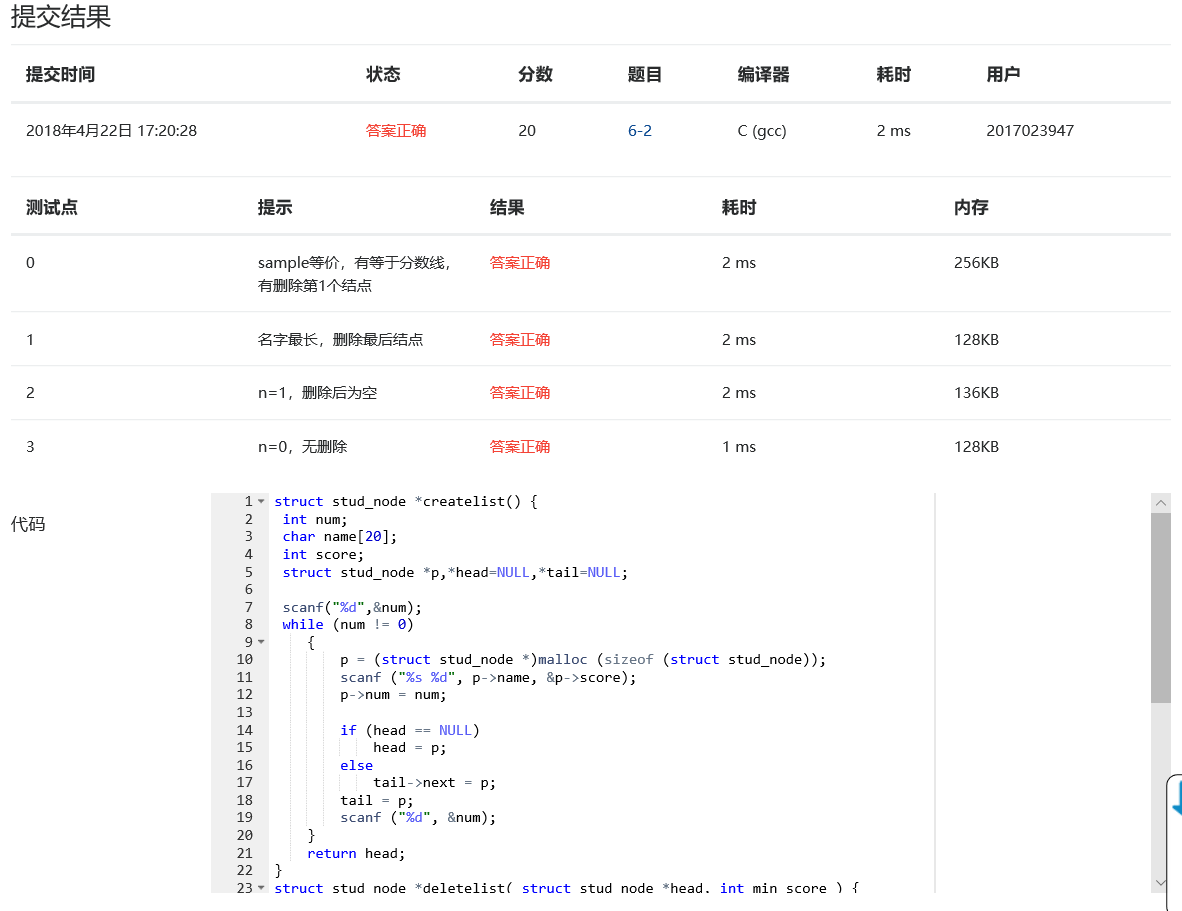6-3 链表拼接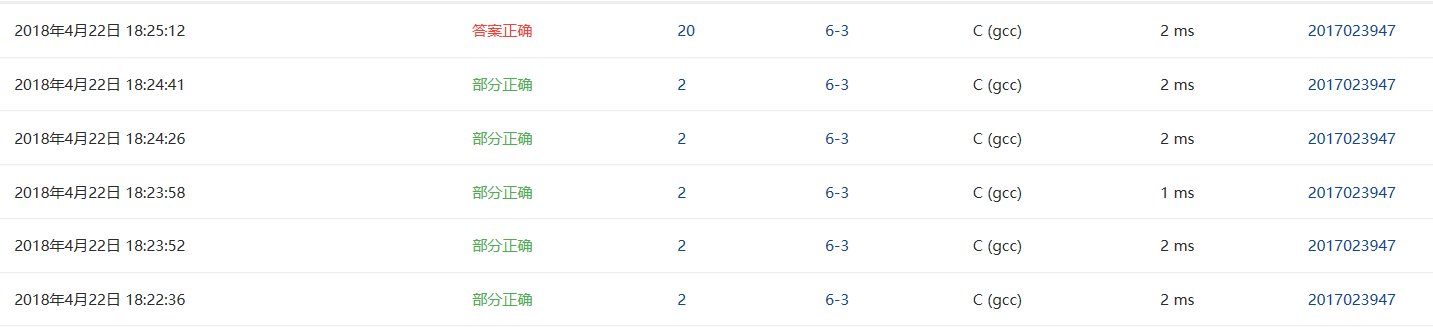1.设计思路
(1)主要描述题目算法。可以用文字描述（不允许使用伪代码），
第一步：
第二步：
......

2.实验代码

struct ListNode *mergelists(struct ListNode *list1, struct ListNode *list2)
{
struct ListNode *p1=list1;
int length=0;
int array;
for(p1=list1;p1!=NULL;p1=p1->next)
{
array[length] = p1->data;
length++;
}
p1=list2;
for(;p1!=NULL;p1=p1->next)
{
array[length] = p1->data;
length++;
}
int i,j,t;
for(i=0;i<length-1;i++)
{
for(j=i+1;j<length;j++)
{
if(array[j]<array[i])
{
t =array[j];
array[j]=array[i];
array[i] = t;
}
}
}
i=0;
while(i<length)
{
q = (struct ListNode *)malloc(sizeof(struct ListNode));
q->data = array[i];
{
} else
{
tail1->next = q;
}
tail1 = q;
tail1->next = NULL;
i++;
}
}  

3.本题调试过程碰到问题及解决办法

错误信息1：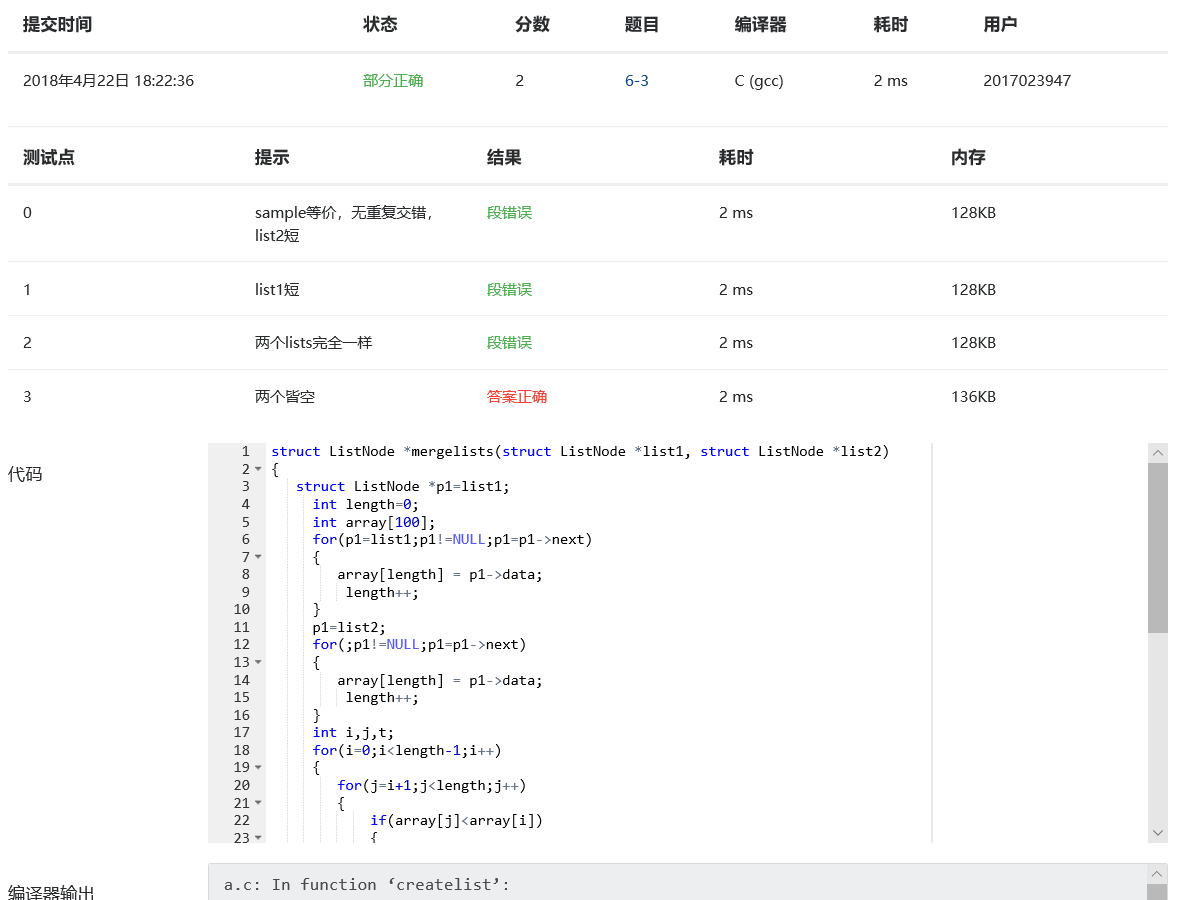错误原因：结尾tail1 = q与tail1->next = NULL的顺序错误
错误信息2：错误原因：最后缺少i++进行循环
改正方法：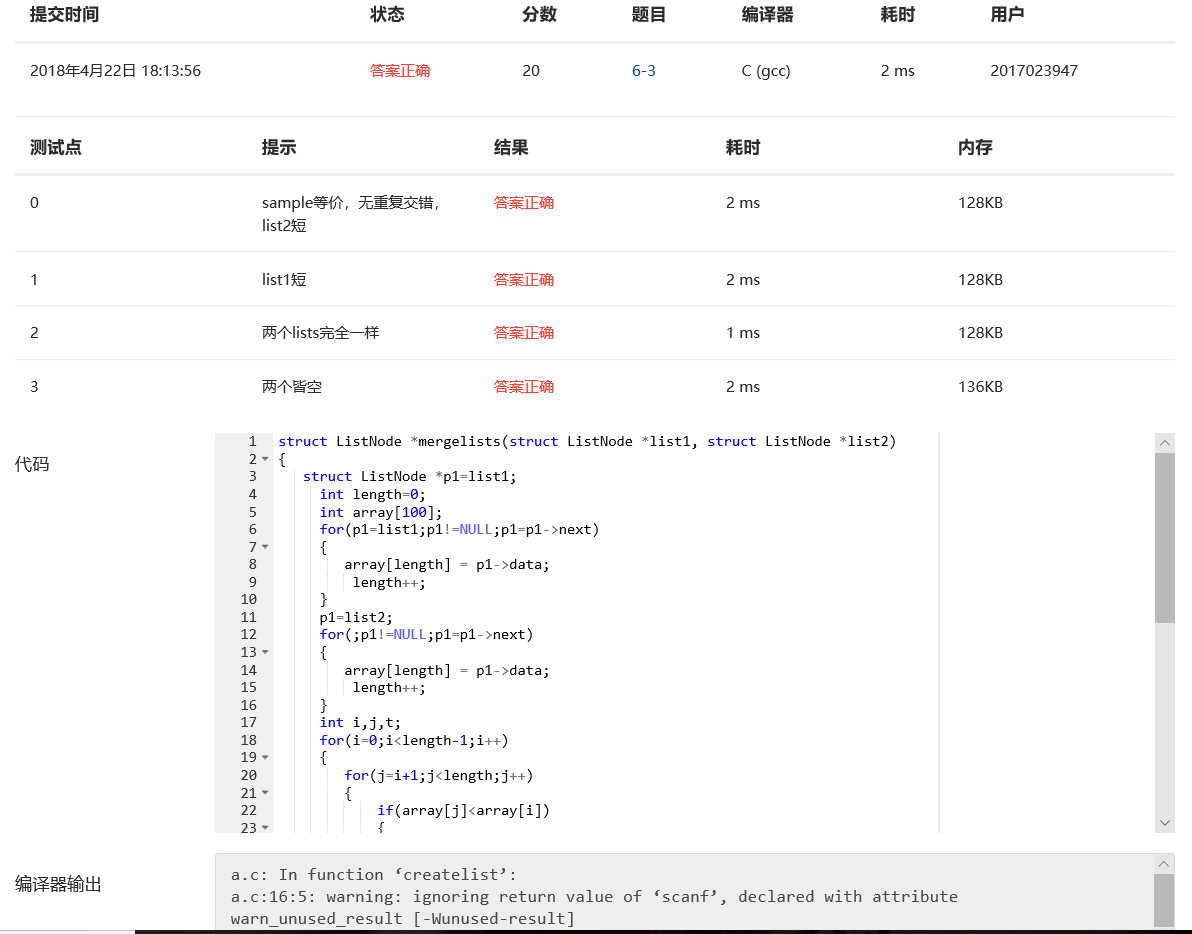#### 要求三、学习总结和进度

1、总结两周里所学的知识点，回答下列问题？

（1）如何理解指针数组，它与指针、数组有何关系？为何可以用二级指针对指针数组进行操作？

数组的各个元素都是指针类型，用于存放内存地址，那么这个数组就是指针数组。二级指针一般定义为“类型名 **变量名”，取得两次地址即可以对指针数组进行操作。

（2）将C高级第三次PTA作业（1）任何一个题目改为使用二级指针对指针数组进行操作。

（3）用指针数组处理多个字符串有何优势？可以直接输入多个字符串给未初始化的指针数组吗？为什么？

节省空间，可自由决定数组长度。不可以，未初始化会将指针存入一个不确定的地址，破坏系统正常工作。

2、将PTA作业的源代码使用git提交到托管平台上，要求给出上传成功截图和你的git地址。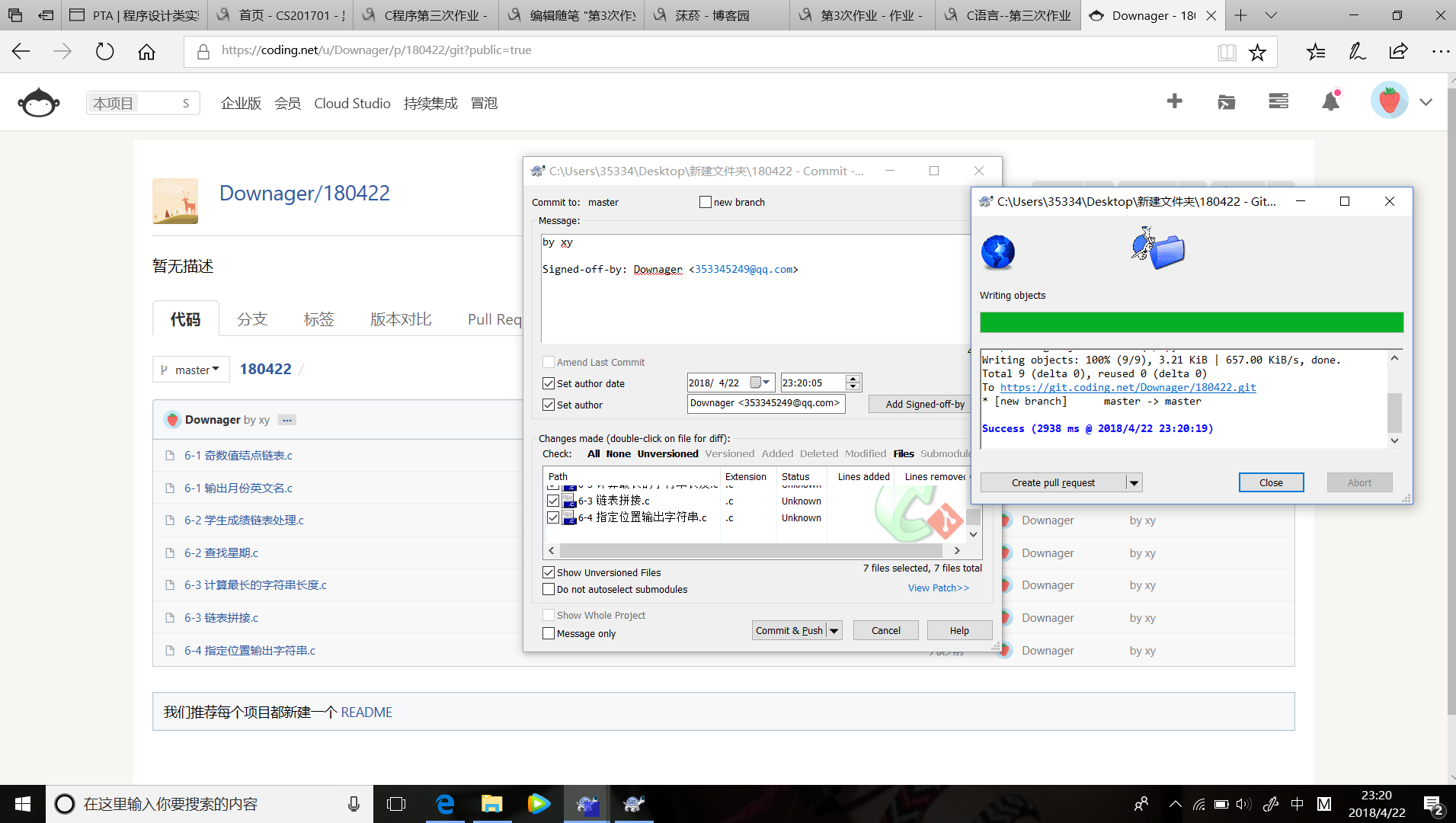3、点评3个同学的本周作业（在作业中给出被点评同学博客的链接），并邀请3名同学点评你的作业，无点评作业(你的作业未被3人点评)/或者没有回复同学或老师的点评都倒扣该题分数。

4、请用表格和折线图呈现你本周（4/9 8:00~4/23 8:00）的代码行数和所用时间、博客字数和所用时间。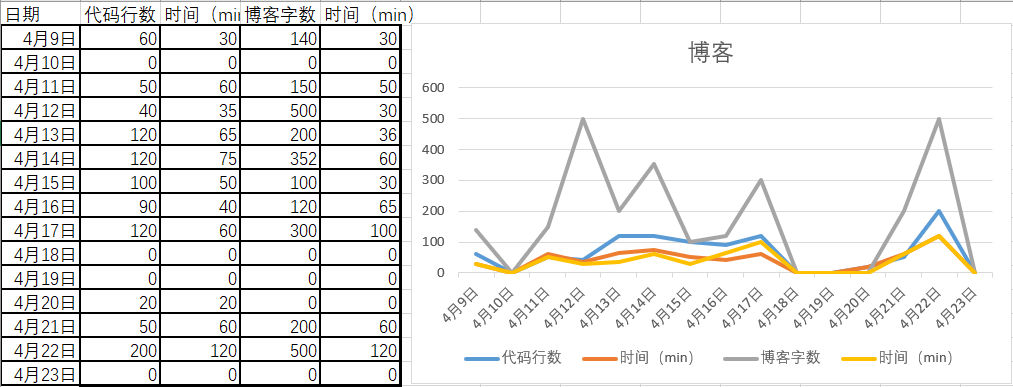posted @ 2018-04-16 22:10  揆空  阅读(377)  评论(9编辑  收藏  举报